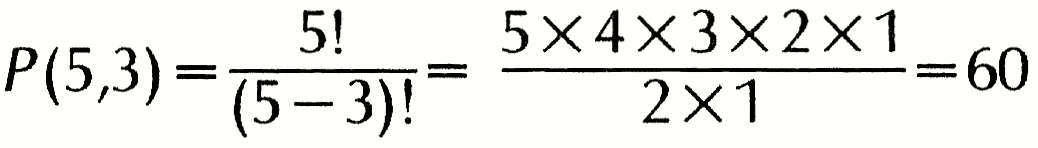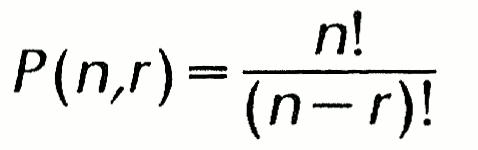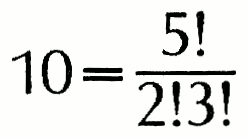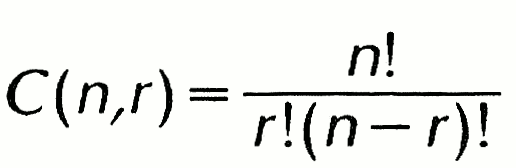Summary Article: probability
From The Columbia Encyclopedia

in mathematics, assignment of a number as a measure of the “chance” that a given event will occur. There are certain important restrictions on such a probability measure. In any experiment there are certain possible outcomes; the set of all possible outcomes is called the sample space of the experiment. To each element of the sample space (i.e., to each possible outcome) is assigned a probability measure between 0 and 1 inclusive (0 is sometimes described as corresponding to impossibility, 1 to certainty). Furthermore, the sum of the probability measures in the sample space must be 1.

Probability of Simple and Compound Events

A simple illustration of probability is given by the experiment of tossing a coin. The sample space consists of one of two outcomes—heads or tails. For a perfectly symmetrical coin, the likely assignment would be 1/2 for heads, 1/2 for tails. The probability measure of an event is sometimes defined as the ratio of the number of outcomes. Thus if weather records for July 1 over a period of 40 years show that the sun shone 32 out of 40 times on July 1, then one might assign a probability measure of 32/40 to the event that the sun shines on July 1.

Probability computed in this way is the basis of insurance calculations. If, out of a certain group of 1,000 persons who were 25 years old in 1900, 150 of them lived to be 65, then the ratio 150/1,000 is assigned as the probability that a 25-year-old person will live to be 65 (the probability of such a person's not living to be 65 is 850/1,000, since the sum of these two measures must be 1). Such a probability statement is of course true only for a group of people very similar to the original group. However, by basing such life-expectation figures on very large groups of people and by constantly revising the figures as new data are obtained, values can be found that will be valid for most large groups of people and under most conditions of life.

In addition to the probability of simple events, probabilities of compound events can be computed. If, for example, A and B represent two independent events, the probability that both A and B will occur is given by the product of their separate probabilities. The probability that either of the two events A and B will occur is given by the sum of their separate probabilities minus the probability that they will both occur. Thus if the probability that a certain man will live to be 70 is 0.5, and the probability that his wife will live to be 70 is 0.6, the probability that they will both live to be 70 is 0.5×0.6=0.3, and the probability that either the man or his wife will reach 70 is 0.5+0.6−0.3=0.8.

Permutations and Combinations

In many probability problems, sophisticated counting techniques must be used; usually this involves determining the number of permutations or combinations. The number of permutations of a set is the number of different ways in which the elements of the set can be arranged (or ordered). A set of 5 books in a row can be arranged in 120 ways, or 5×4×3×2×1=5!=120 (the symbol 5!, denoting the product of the integers from 1 to 5, is called factorial 5). If, from the five books, only three at a time are used, then the number of permutations is 60, orIn general the number of permutations of n things taken r at a time is given byOn the other hand, the number of combinations of 3 books that can be selected from 5 books refers simply to the number of different selections without regard to order. The number in this case is 10:In general, the number of combinations of n things taken r at a time isStatistical Inference

The application of probability is fundamental to the building of statistical forms out of data derived from samples (see statistics). Such samples are chosen by predetermined and arbitrary selection of related variables and arbitrary selection of intervals for sampling; these establish the degree of freedom. Many courses are given in statistical method. Elementary probability considers only finite sample spaces; advanced probability by use of calculus studies infinite sample spaces. The theory of probability was first developed (c.1654) by Blaise Pascal, and its history since then involves the contributions of many of the world's great mathematicians.

Bibliography
• See , Probability and Measure (1979);.
• , The Emergence of Probability (1984, rev. ed. 2006);.
• , Probability (1986);.
• , Introduction to Probability (1988);.
• , Introduction to Probability Theory (1989).The Columbia Encyclopedia, © Columbia University Press 2018

### Related Articles

##### Full text Article PROBABILITY
Encyclopedia of Empiricism

In the history of empiricism, probability has been a central and distinctive concern, but the understanding of it has proved elusive. It is, of...

##### Full text Article probability
Dictionary of World Philosophy

From the Latin probabilitas, and this from the Latin probabilis (“capable of standing a test”) and the particle -tas, where probabilis ...

##### Full text Article Probability
Reader's Guide to the History of Science

Dale Andrew I. , A History of Inverse Probability: From Thomas Bayes to Karl Pearson , New York : Springer , 1991 Daston ...

See more from Credo